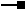# IRasterColormap2 Interface

## Members

Name DescriptionBin Translates pixel values into integers to index them into the colormap.BinFunction The Bin function that maps pixel value into colormap index.BlueValues Array of blue ratios as doubles between 0.0 and 1.0.Colors Array of colors as OLE_​COLORs.GreenValues Array of green ratios as doubles between 0.0 and 1.0.LUT The lookup table (OBJECTID, VALUE) for remaping from index to pixel value.RedValues Array of red ratios as doubles between 0.0 and 1.0.RemappedBlueValues The remapped Blue color component values using LUT from index to value (OBJECTID, VALUE).RemappedColors The remapped colors using LUT from index to value (OBJECTID, VALUE).RemappedGreenValues The remapped Green color component values using LUT from index to value (OBJECTID, VALUE).RemappedRedValues The remapped Red color component values using LUT from index to value (OBJECTID, VALUE).

### IRasterColormap2.BinFunction Property

The Bin function that maps pixel value into colormap index.

``````Public ReadOnly Property BinFunction As IBinFunction
``````
``````public IBinFunction BinFunction {get;}
``````

### IRasterColormap2.LUT Property

The lookup table (OBJECTID, VALUE) for remaping from index to pixel value.

``````Public WriteOnly Property LUT
``````
``````public void LUT {set;}
``````

#### Description

Set the lookup table to map index to raster pixel value.

### IRasterColormap2.RemappedBlueValues Property

The remapped Blue color component values using LUT from index to value (OBJECTID, VALUE).

``````Public ReadOnly Property RemappedBlueValues As Object
``````
``````public object RemappedBlueValues {get;}
``````

### IRasterColormap2.RemappedColors Property

The remapped colors using LUT from index to value (OBJECTID, VALUE).

``````Public ReadOnly Property RemappedColors As Object
``````
``````public object RemappedColors {get;}
``````

### IRasterColormap2.RemappedGreenValues Property

The remapped Green color component values using LUT from index to value (OBJECTID, VALUE).

``````Public ReadOnly Property RemappedGreenValues As Object
``````
``````public object RemappedGreenValues {get;}
``````

### IRasterColormap2.RemappedRedValues Property

The remapped Red color component values using LUT from index to value (OBJECTID, VALUE).

``````Public ReadOnly Property RemappedRedValues As Object
``````
``````public object RemappedRedValues {get;}
``````

## Inherited Interfaces

Interfaces Description